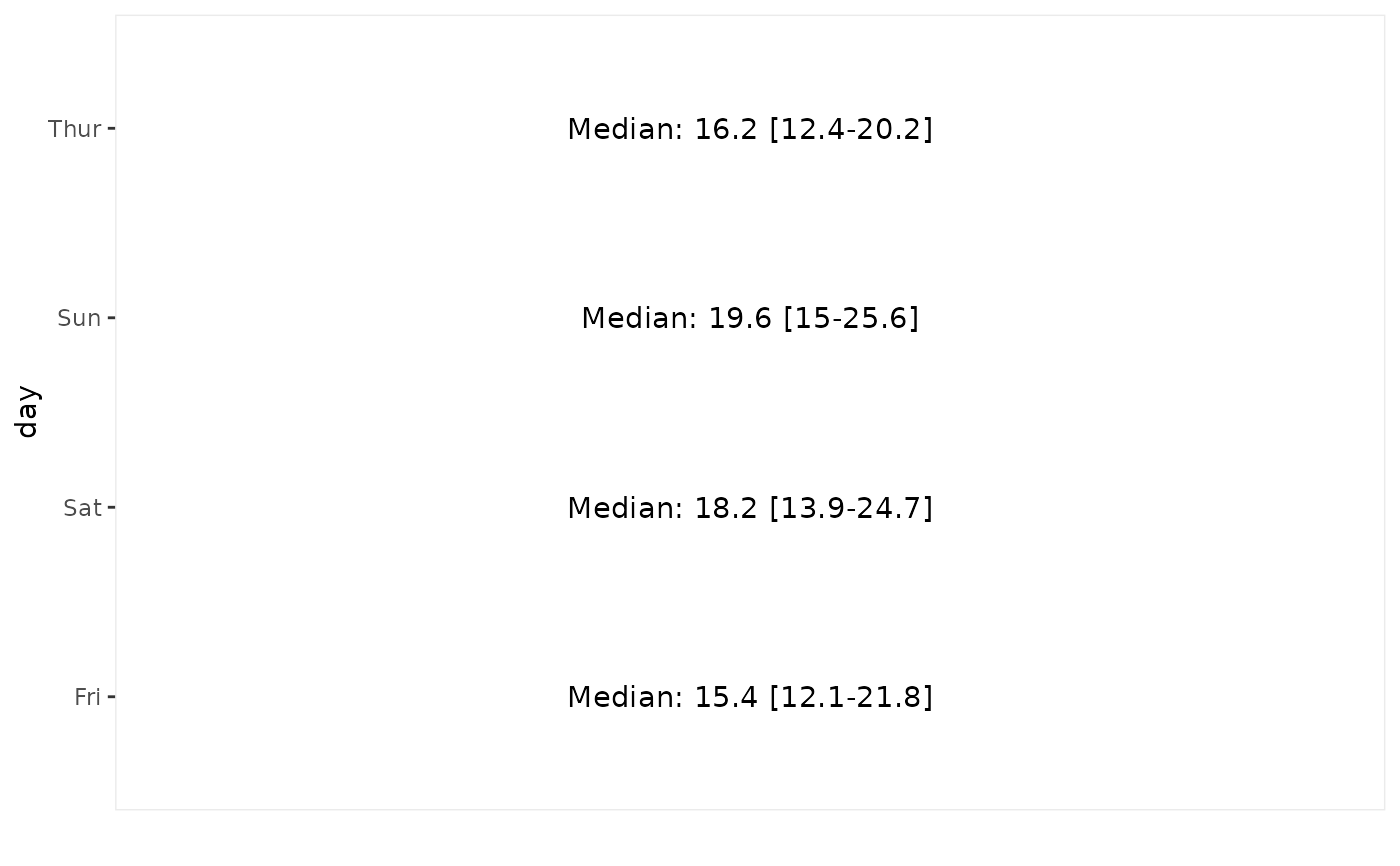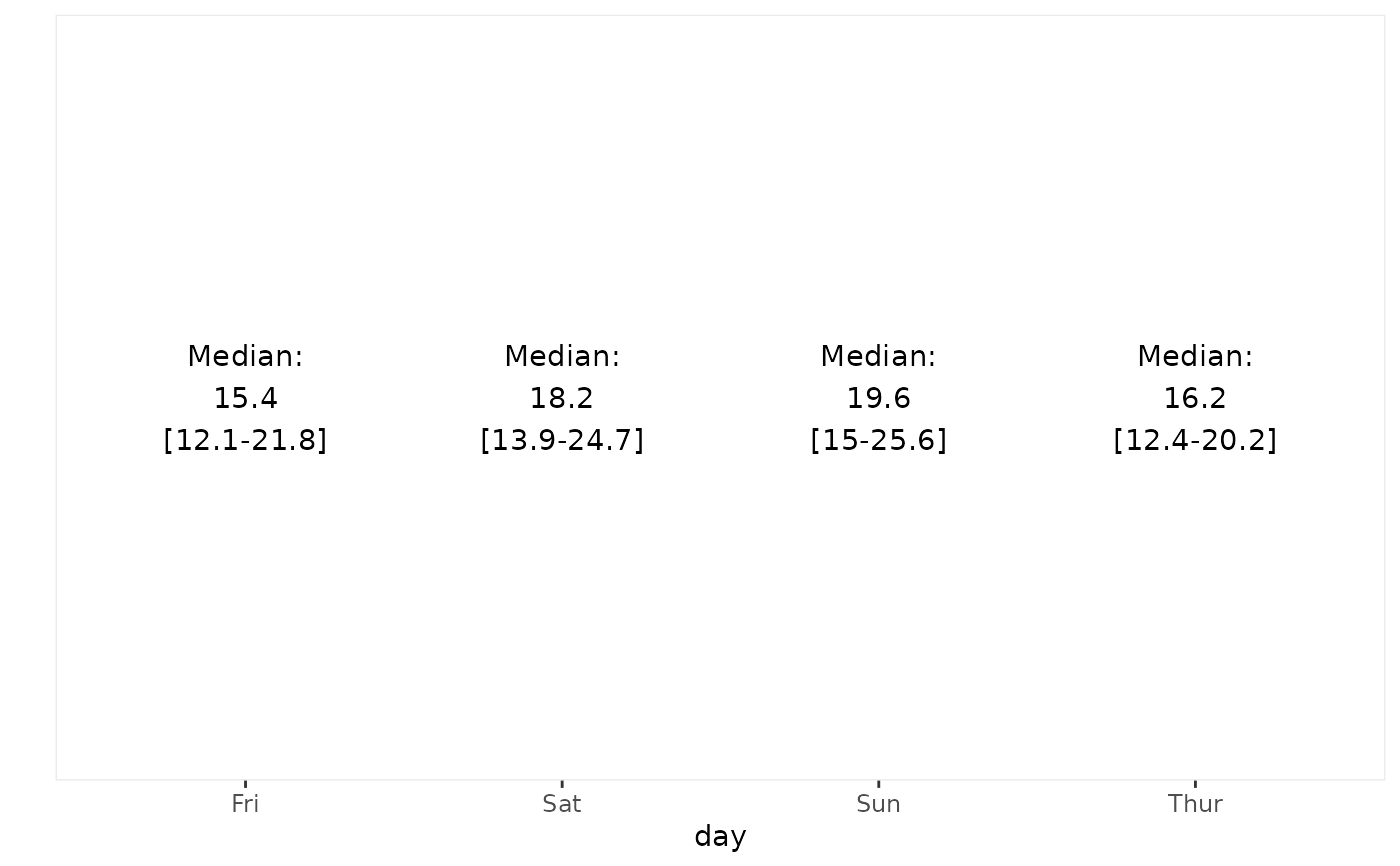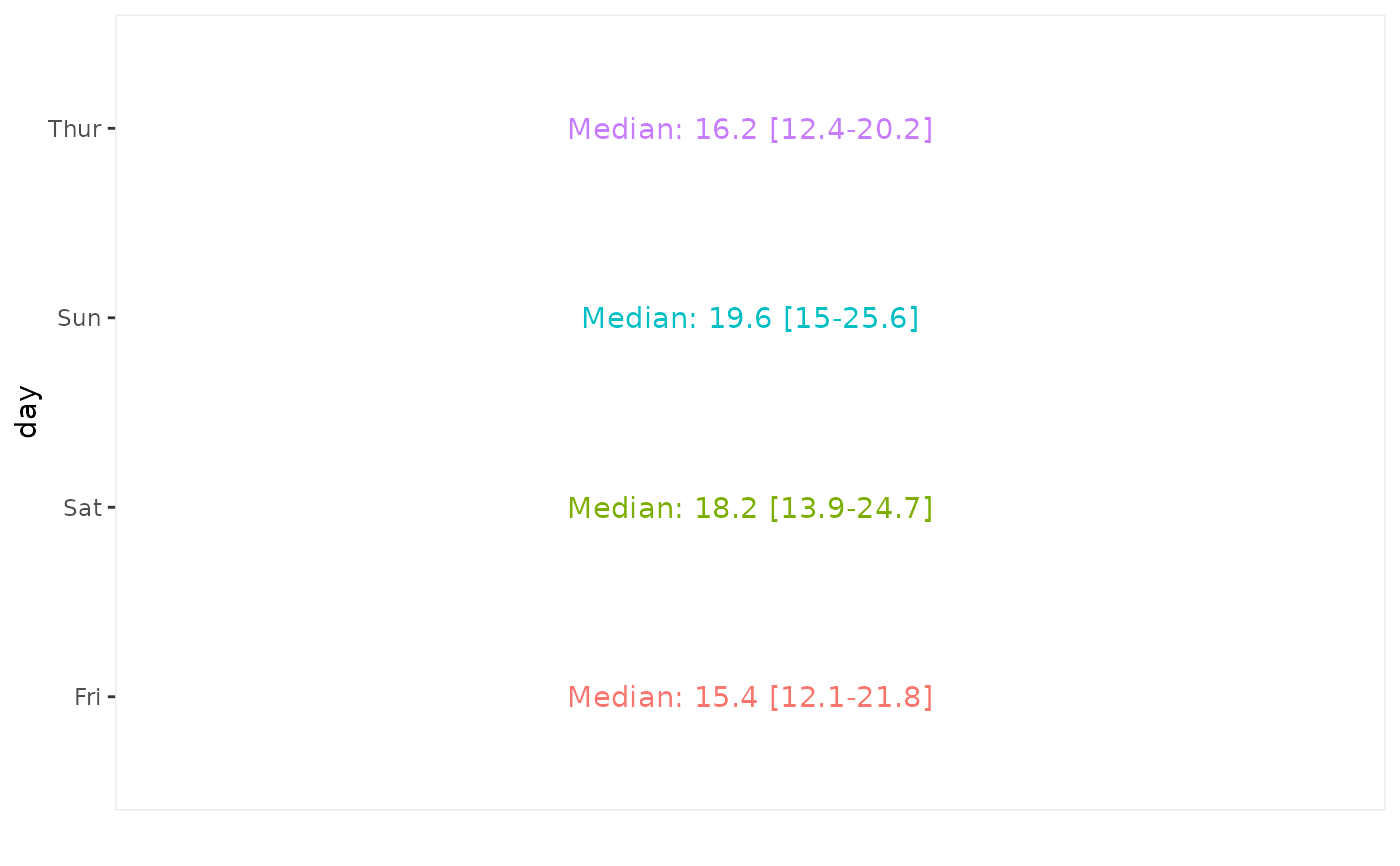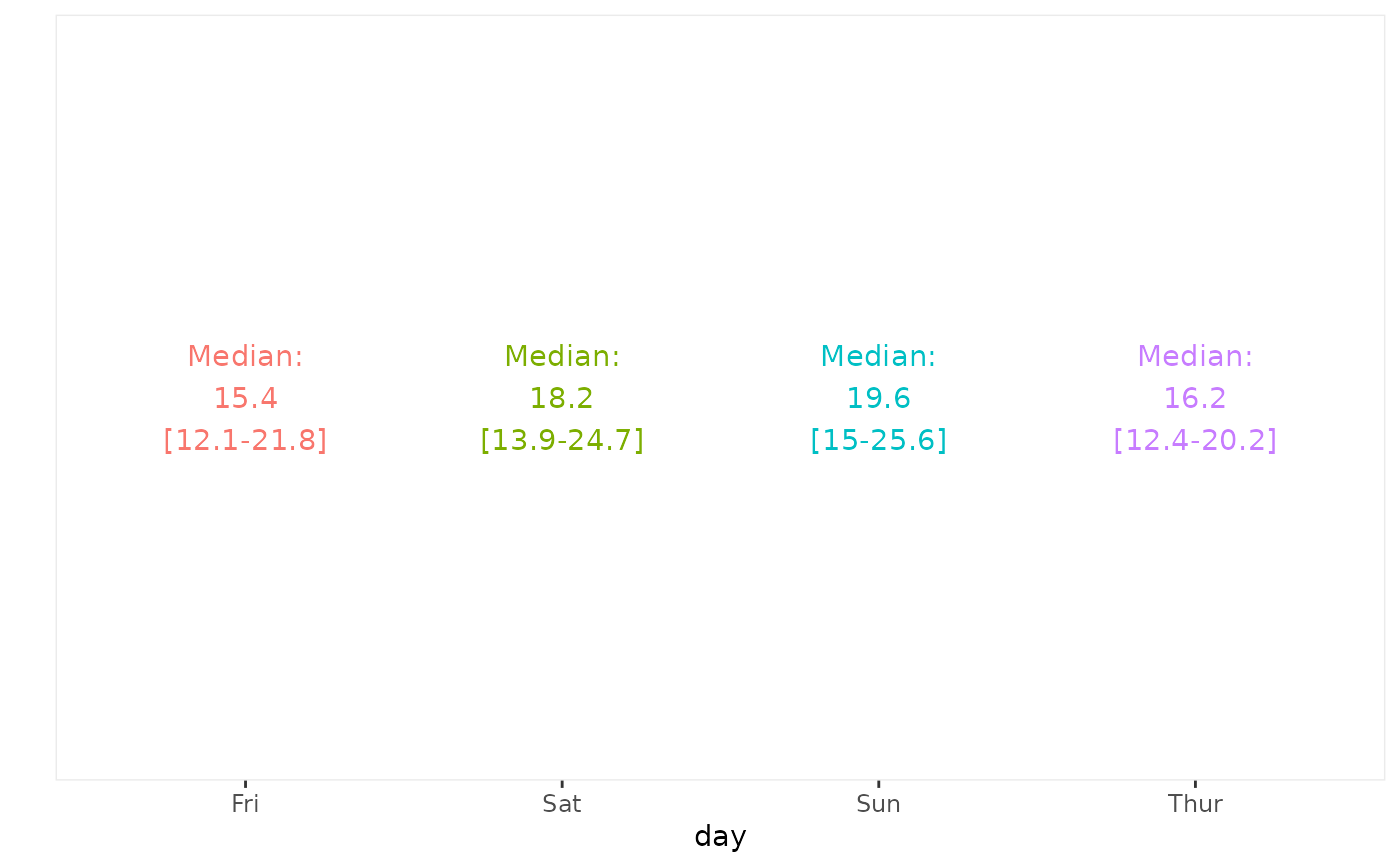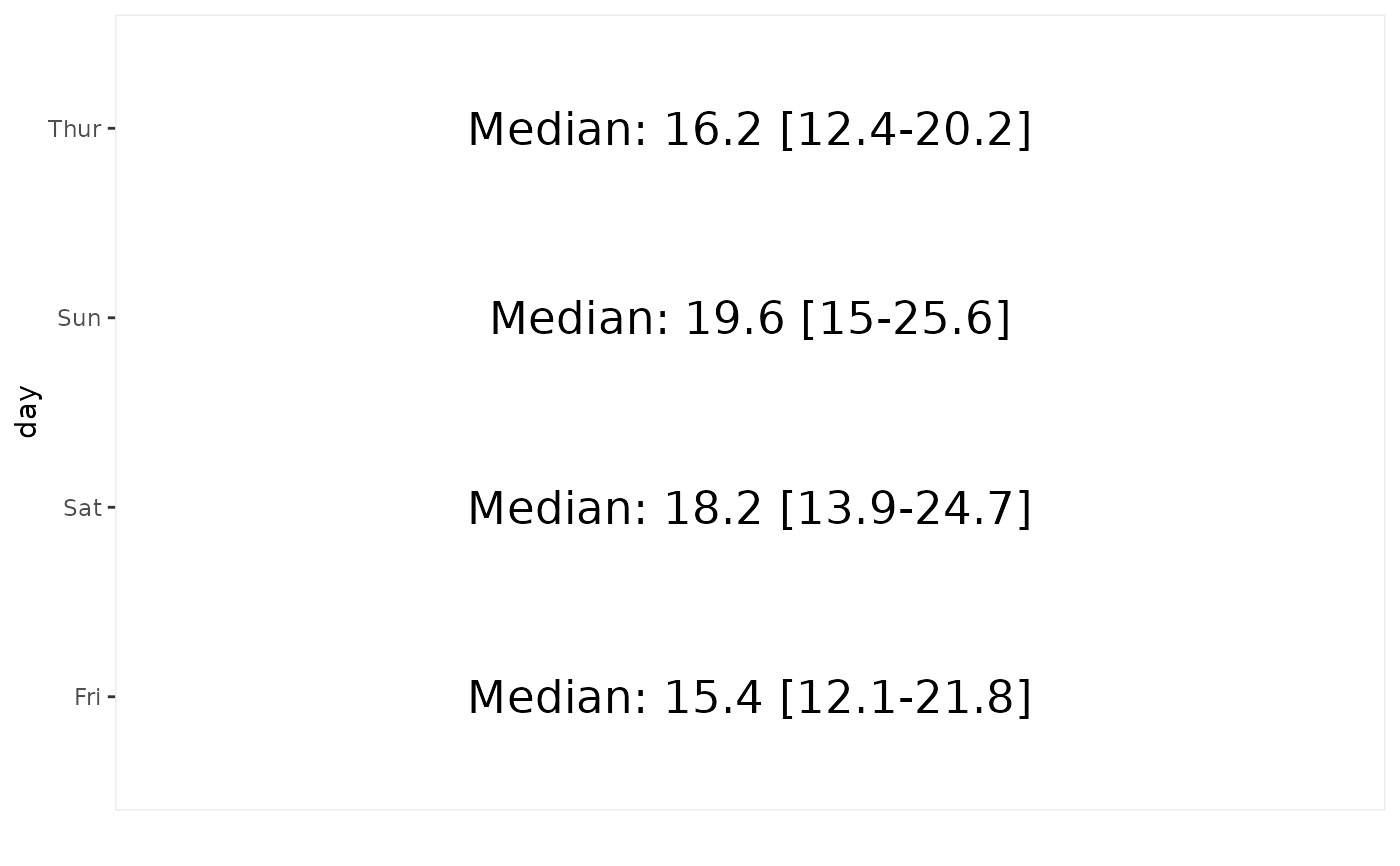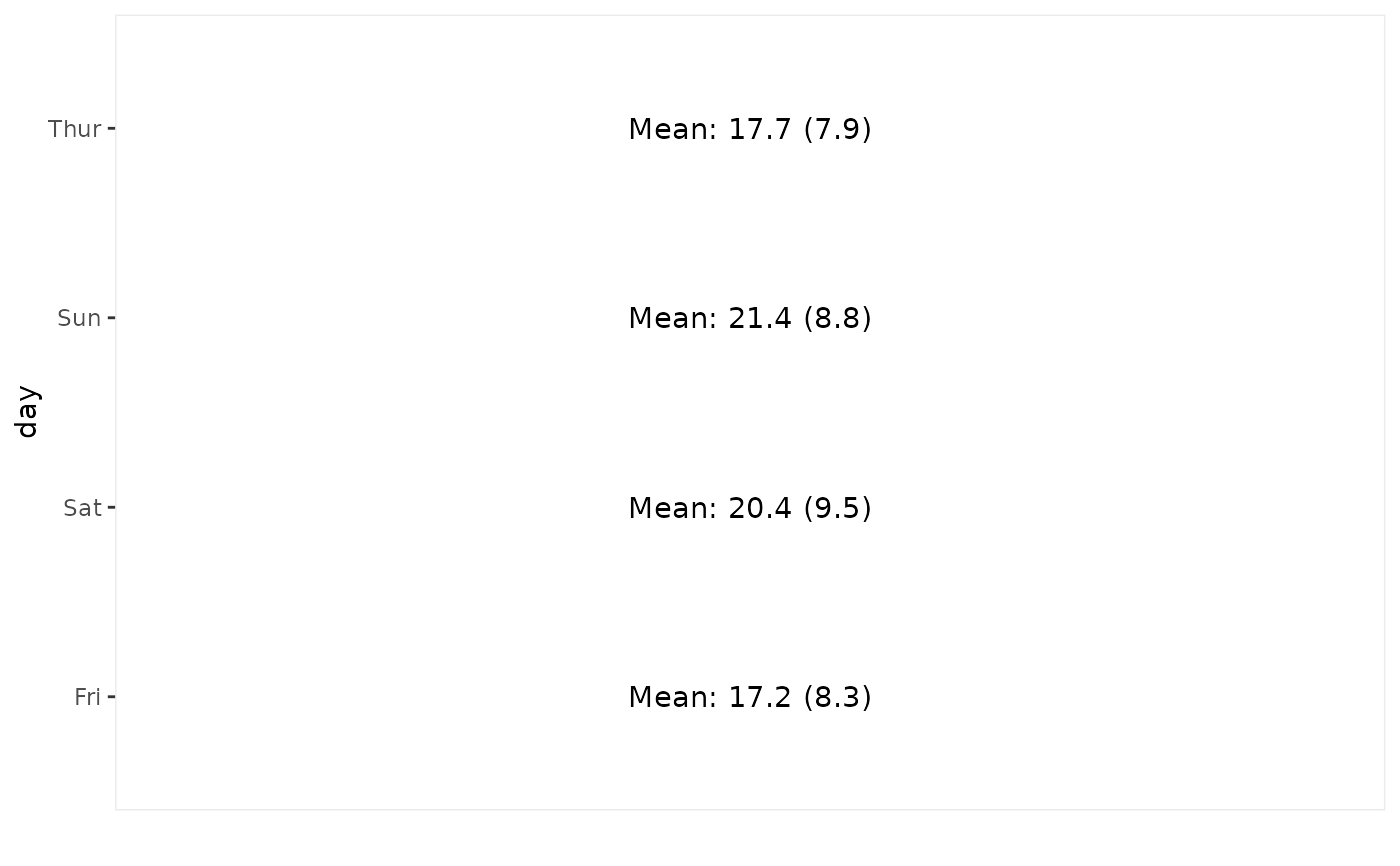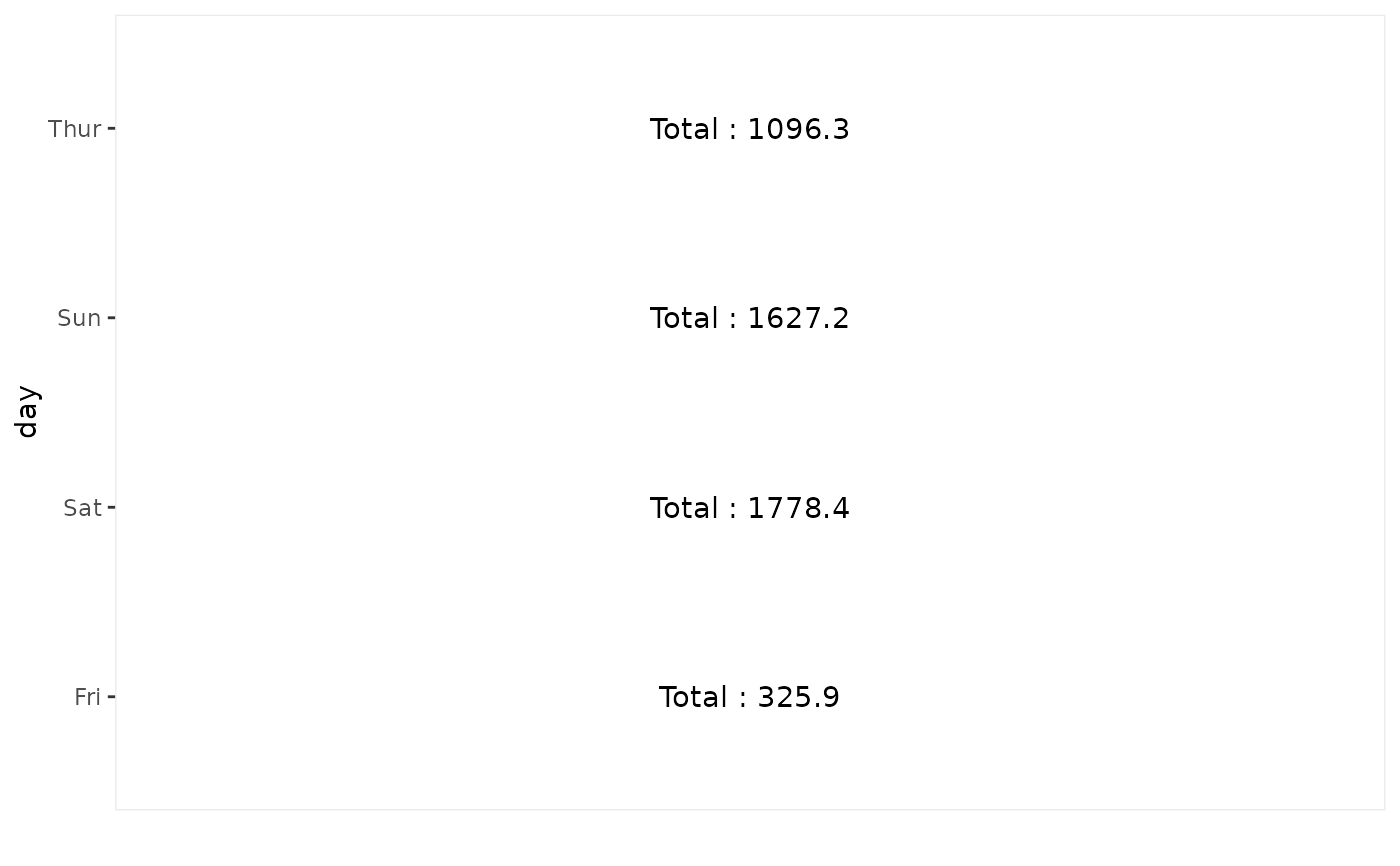Display summary statistics of a continuous variable for each value of a discrete variable.

ggally_summarise_by(
data,
mapping,
text_fn = weighted_median_iqr,
text_fn_vertical = NULL,
...
)

weighted_median_iqr(x, weights = NULL)

weighted_mean_sd(x, weights = NULL)

## Arguments

data data set using aesthetics being used function that takes an x and weights and returns a text string function that takes an x and weights and returns a text string, used when x is discrete and y is continuous. If not provided, will use text_fn, replacing spaces by carriage returns. other arguments passed to geom_text(...) a numeric vector an optional numeric vectors of weights. If NULL, equal weights of 1 will be taken into account.

## Details

weighted_median_iqr computes weighted median and interquartile range.

weighted_mean_sd computes weighted mean and standard deviation.

## Author

Joseph Larmarange

## Examples

# Small function to display plots only if it's interactive
p_ <- GGally::print_if_interactive

if (require(Hmisc)) {
data(tips, package = "reshape")
p_(ggally_summarise_by(tips, mapping = aes(x = total_bill, y = day)))
p_(ggally_summarise_by(tips, mapping = aes(x = day, y = total_bill)))

# colour is kept only if equal to the discrete variable
p_(ggally_summarise_by(tips, mapping = aes(x = total_bill, y = day, color = day)))
p_(ggally_summarise_by(tips, mapping = aes(x = total_bill, y = day, color = sex)))
p_(ggally_summarise_by(tips, mapping = aes(x = day, y = total_bill, color = day)))

# custom text size
p_(ggally_summarise_by(tips, mapping = aes(x = total_bill, y = day), size = 6))

# change statistic to display
p_(ggally_summarise_by(tips, mapping = aes(x = total_bill, y = day), text_fn = weighted_mean_sd))

# custom stat function
weighted_sum <- function(x, weights = NULL) {
if (is.null(weights)) weights <- 1
paste0("Total : ", round(sum(x * weights, na.rm = TRUE), digits = 1))
}
p_(ggally_summarise_by(tips, mapping = aes(x = total_bill, y = day), text_fn = weighted_sum))
}
#>     format.pval, units